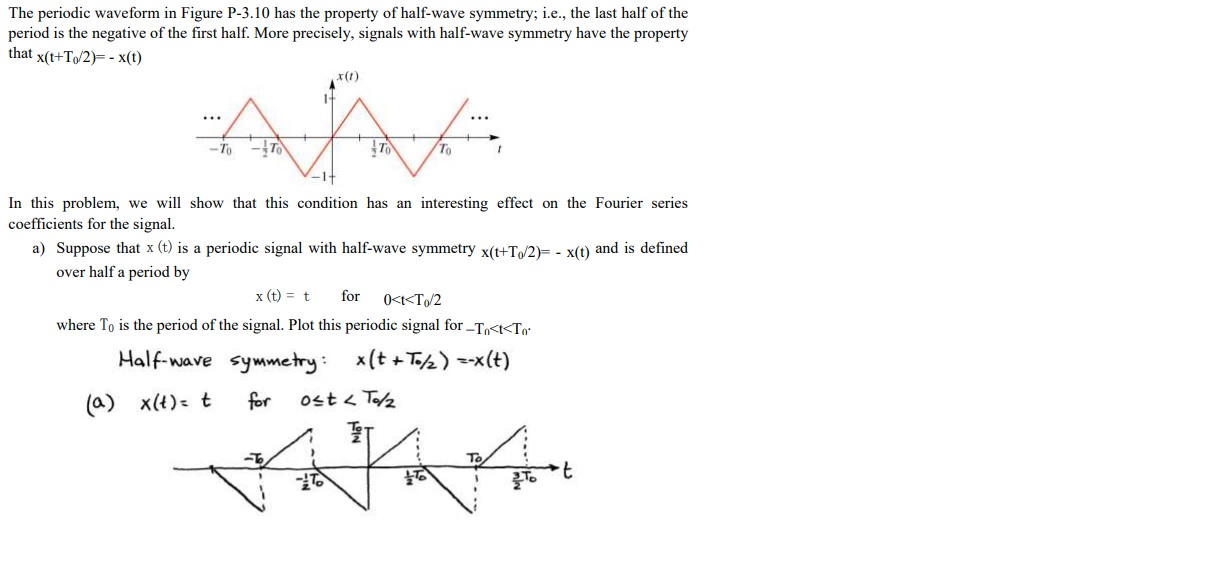# Question The periodic waveform in Figure P-3.10 has the property of half-wave symmetry; i.e., the last half of the period is the negative of the first half. More precisely, signals with half-wave symmetry have the property that X(t+T/2)= - X(t) In this problem, we will show that this condition has an interesting effect on the Fourier series coefficients for the The periodic waveform in Figure P-3.10 has the property of half-wave symmetry; i.e., the last half of the period is the negative of the first half. More precisely, signals with half-wave symmetry have the property that X(t+T/2)= - X(t) In this problem, we will show that this condition has an interesting effect on the Fourier series coefficients for the signal. a) Suppose that x (t) is a periodic signal with half-wave symmetry x(t+T/2) - X(t) and is defined over half a period by x(t) = t for <<T/2 where To is the period of the signal. Plot this periodic signal for -T,<<<T, Half-wave symmetry: *(t + To/2) =-*(t) (a) X(t)= t for ost < TolzT9OTRD The Asker · Electrical EngineeringI need a step by step explanation for this solution.

Transcribed Image Text: The periodic waveform in Figure P-3.10 has the property of half-wave symmetry; i.e., the last half of the period is the negative of the first half. More precisely, signals with half-wave symmetry have the property that X(t+T/2)= - X(t) In this problem, we will show that this condition has an interesting effect on the Fourier series coefficients for the signal. a) Suppose that x (t) is a periodic signal with half-wave symmetry x(t+T/2) - X(t) and is defined over half a period by x(t) = t for <
More
Transcribed Image Text: The periodic waveform in Figure P-3.10 has the property of half-wave symmetry; i.e., the last half of the period is the negative of the first half. More precisely, signals with half-wave symmetry have the property that X(t+T/2)= - X(t) In this problem, we will show that this condition has an interesting effect on the Fourier series coefficients for the signal. a) Suppose that x (t) is a periodic signal with half-wave symmetry x(t+T/2) - X(t) and is defined over half a period by x(t) = t for <